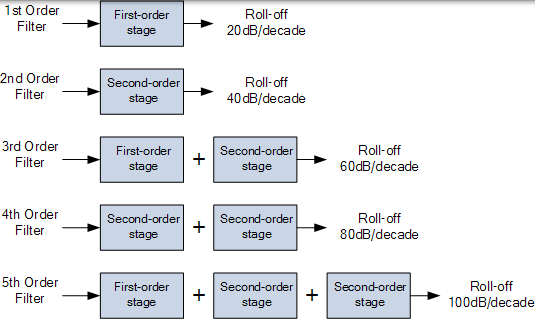# 有源高通滤波器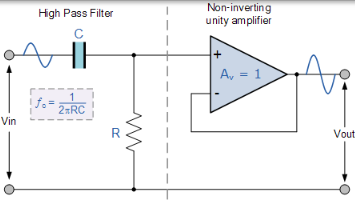### 一阶高通滤波器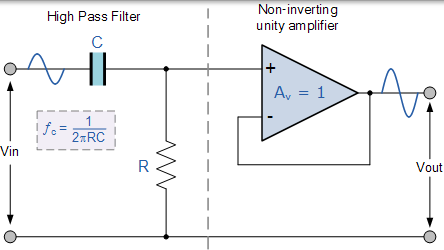### 典型运算放大器的频率响应曲线## 有源高通滤波器

### 具有放大功能的有源高通滤波器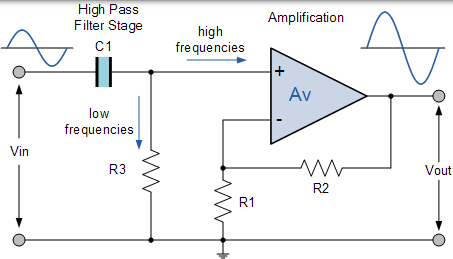### 增益有源高通滤波器

$$\text { Voltage Gain, } ( \mathrm { Av } ) = \frac { \mathrm { Vout } } { \mathrm { Vin } } = \frac { \mathrm { A } _ { \mathrm { F } } \left( \frac { f } { f \mathrm { c } } \right) } { \sqrt { 1 + \left( \frac { f } { f \mathrm { c } } \right) ^ { 2 } } }$$

• AF =滤波器的通带增益，（ 1 + R2 / R1 ）
• ƒ = 输入信号的频率，单位为赫兹，（Hz）
• ƒc = 以赫兹为单位的截止频率，（Hz）

1. 在非常低的频率下，ƒ < ƒc 时，$\frac { \mathrm { Vout } } { \mathrm { Vin } } < \mathrm { A } _ { \mathrm { F } }$
2. 在截止频率，ƒ = ƒc 时，$\frac { ) Vout ( } { V ) in ( } = \frac { A _ { F } } { \sqrt { 2 } } = 0.707 \mathrm { A } _ { \mathrm { F } }$
3. 在非常高的频率下，ƒ > ƒc 时， \frac { \mathrm { Vout } } { \mathrm { Vin } } \cong \mathrm { A } _ { \mathrm { F } }

### 电压增益幅度（dB）

$$\begin{array} { c } { \mathrm { Av } ( \mathrm { dB } ) = 20 \log _ { 10 } \left( \frac { \mathrm { Vout } } { \mathrm { Vout } } \right) } \\ { \therefore - 3 \mathrm { dB } = 20 \log _ { 10 } \left( 0.707 \frac { \mathrm { Vout } } { \mathrm { Vin } } \right) } \end{array}$$

$$f _ { C } = \frac { 1 } { 2 \pi R C } \textrm {Hz}$$

$$\text { Phase Shift } \phi = \tan ^ { - 1 } \left( \frac { 1 } { 2 \pi / R C } \right)$$

### 反相运算放大器电路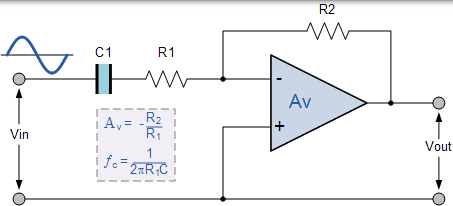### 频率响应曲线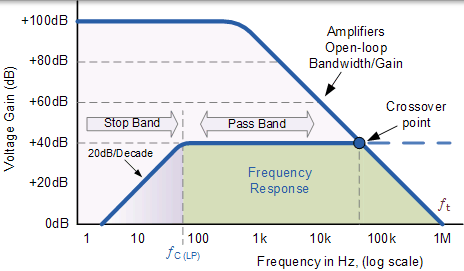## 有源高通滤波器示例 No1

$$\mathrm { R } = \frac { 1 } { 2 \pi f _ { \mathrm { c } } \mathrm { C } } = \frac { 1 } { 2 \pi .1000 .10 \times 10 ^ { - 9 } } = 15.92 \mathrm { kS }$$

$$\mathrm { A } _ { \mathrm { F } } = 1 + \frac { \mathrm { R } _ { 2 } } { \mathrm { R } _ { 1 } } , \quad \therefore 2 = 1 + \frac { \mathrm { R } _ { 2 } } { \mathrm { R } _ { 1 } } \quad \text { and } \quad \frac { \mathrm { R } _ { 2 } } { \mathrm { R } _ { 1 } } = 1$$

$$\text { Voltage Gain, } ( \mathrm { Av } ) = \frac { \text { Vout } } { \mathrm { Vin } } = \frac { \mathrm { A } _ { \mathrm { F } } \left( \frac { f } { f \mathrm { c } } \right) } { \sqrt { 1 + \left( \frac { f } { f \mathrm { c } } \right) ^ { 2 } } }$$

100 0.20 -14.02
200 0.39 -8.13
500 0.89 -0.97
800 1.25 1.93
1000 1.41 3.01
3000 1.90 5.56
5000 1.96 5.85
10000 1.99 5.98
50000 2.00 6.02
100000 2.00 6.02

### 例子的频率响应波特图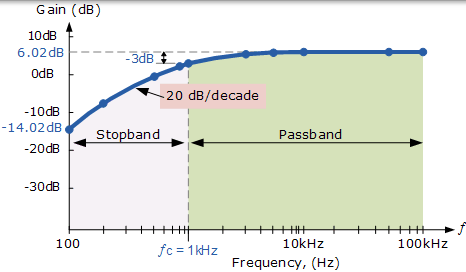## 二阶高通有源滤波器

### 二阶有源高通滤波器电路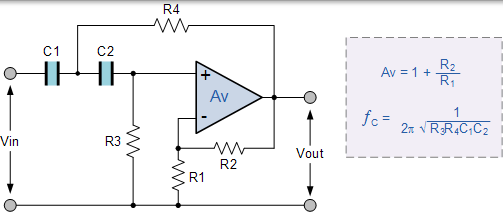### 级联有源高通滤波器